# 【自学】深度学习入门 基于python的理论与实现 LESSON8 ＜误差反向传播法2＞

Rachel MuZy · 收录于 2023-11-21 05:03:41 · source URL

1.ReLU层

2.Sigmoid层

1. Affine层

## 一、布尔值的理解

``````import numpy as np

a = np.array([[1, 2, -3], [2, 3, 4]])
c = (a<=0)
b = a.copy()
b[c] = 0
print(b)
print(c)``````

``````[[1 2 0]
[2 3 4]]
[[False False  True]
[False False False]]``````

（1）c = (a<=0) 表示如果a的值有<=0的存在，就是true，否则是false。此时c为布尔值组成的矩阵

（2）b = a.copy() 表示b = a

（3）b[c] = 0 表示如果c矩阵中有true，则b矩阵的对应位置输出为0，否则输出为b矩阵的原值。

## 二、激活函数层的实现

### 1.ReLU层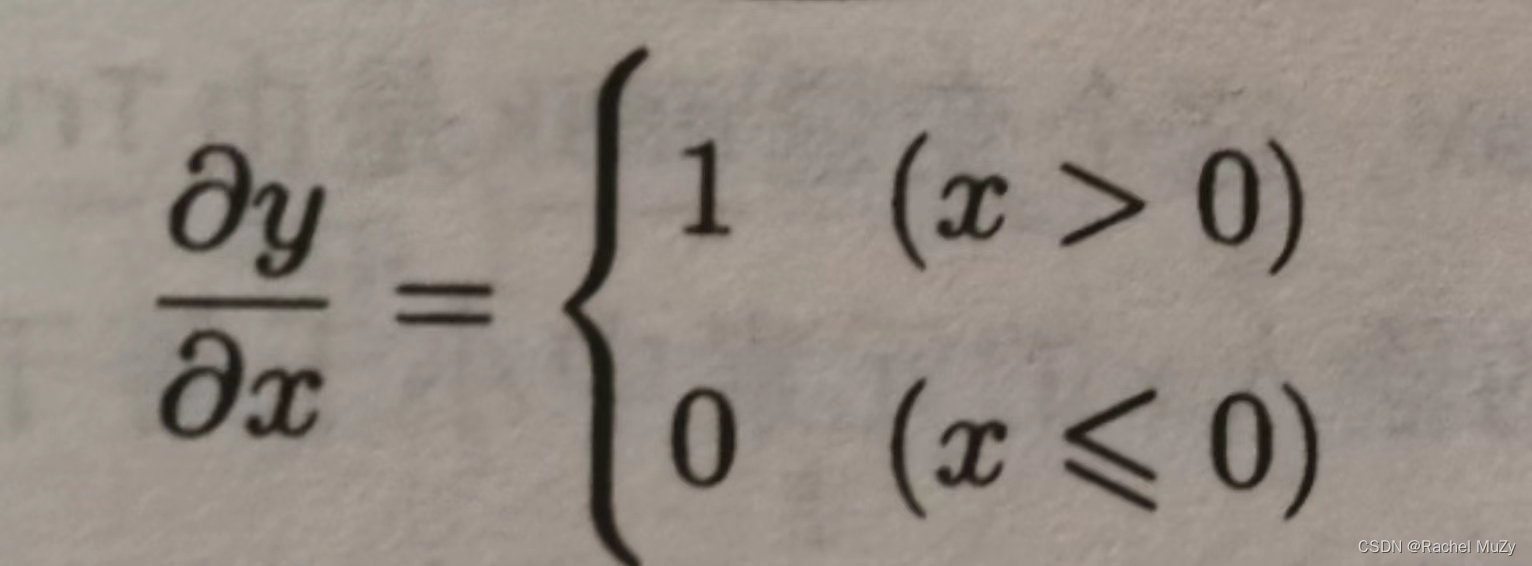代码：

``````import numpy as np

class ReLU():
def __init__(self):

def forward(self, x):
out = x.copy()

return out

def backward(self, dout):
dx = dout

return dx``````

``````[[1. 0.]
[0. 3.]]``````

``````import numpy as np

class ReLU():
def __init__(self):

def forward(self, x):
buer_k = []
for i in x:
for k in i:
if k <= 0:
k = 0
buer_k.append(k)
buer_k = np.array(buer_k)
out = buer_k.reshape((2,2))
return out

def backward(self, dout):
dx = dout

return dx

a = np.array([[1.0, -0.5], [-2.0, 3.0]])
relu = ReLU()
out = relu.forward(a)
print(out)``````

``````[[1. 0.]
[0. 3.]]``````

### 2.Sigmoid层

sigmoid函数的反向传播：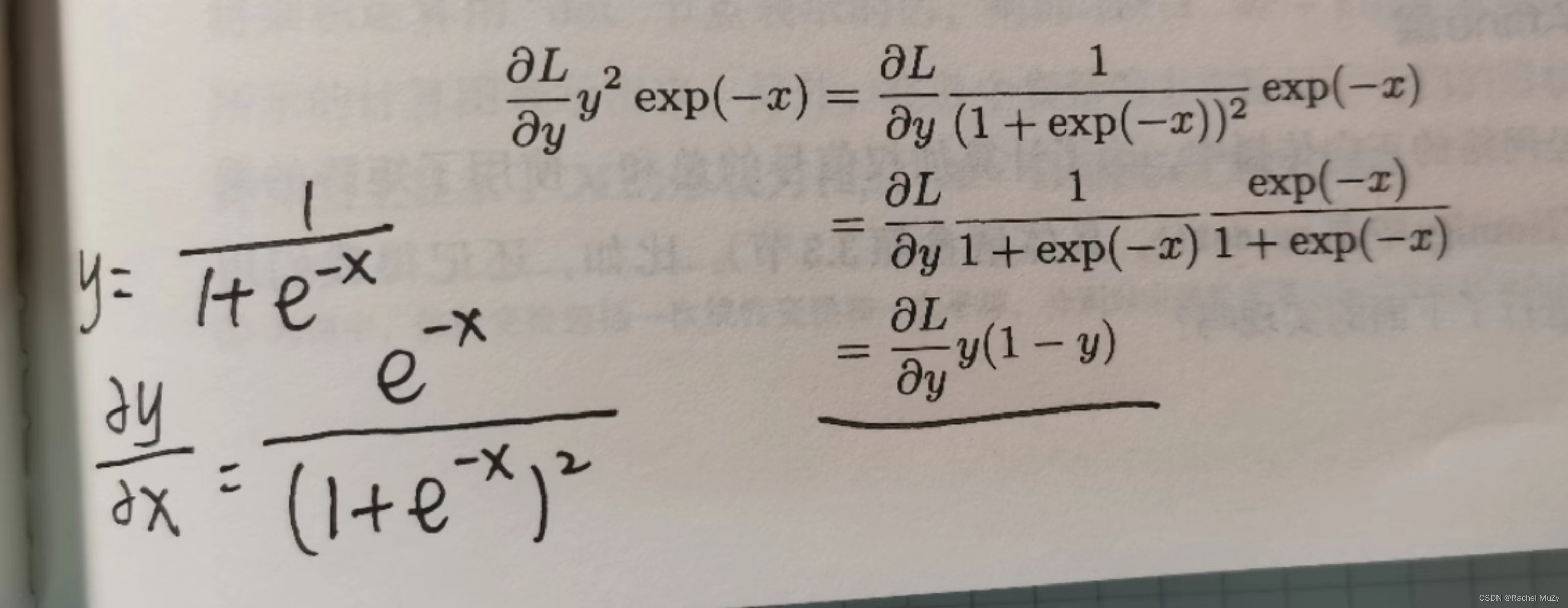L表示损失函数。损失函数的输入是y（神经网络的输出），t（监督数据）。神经网络的梯度是 L 对 W （权重）的偏导，Y = np.dot(X, W) + B，Y再经过激活函数后的值，才是损失函数的输入y。因此想要反向传播，要先求激活函数的导。而上图中的x指的是np.dot(X, W) + B，并不是最原始的输入数据。

``````import numpy as np

class Sigmoid():
def __init__(self):
self.out = None

def forward(self, x):
out = 1 / (1 + np.exp(-x))
self.out = out
return out

def backward(self, dout):
dx = dout * self.out * (1 - self.out)
#并不理解dout表示的是什么，是损失函数对输出的偏导吗？但是此处用dout，是对输出求导。感到非常迷惑
return dx``````

## 三、Affine/Softmax层的实现

### 1. Affine层

Y = np.dot(X, W) + B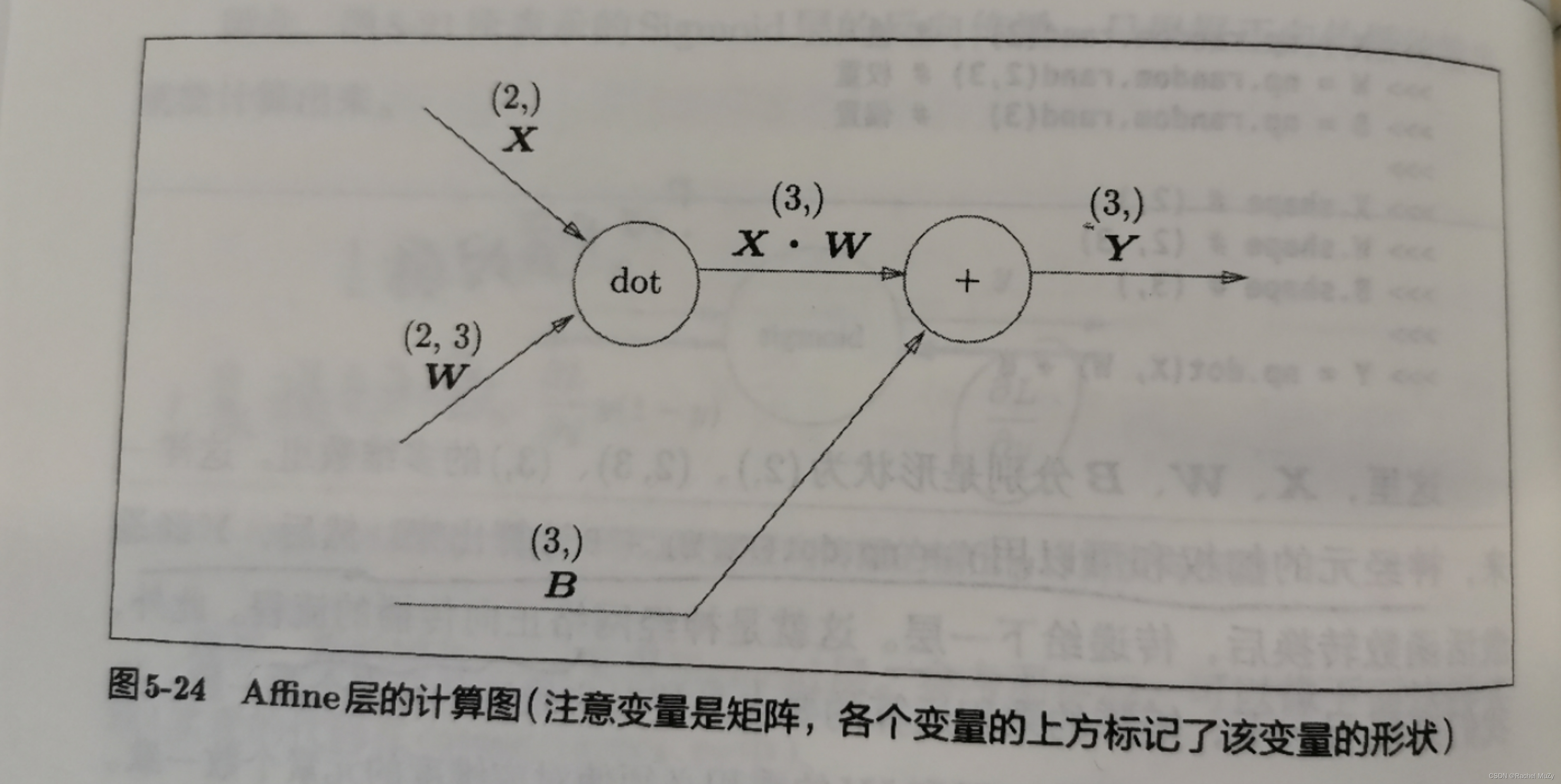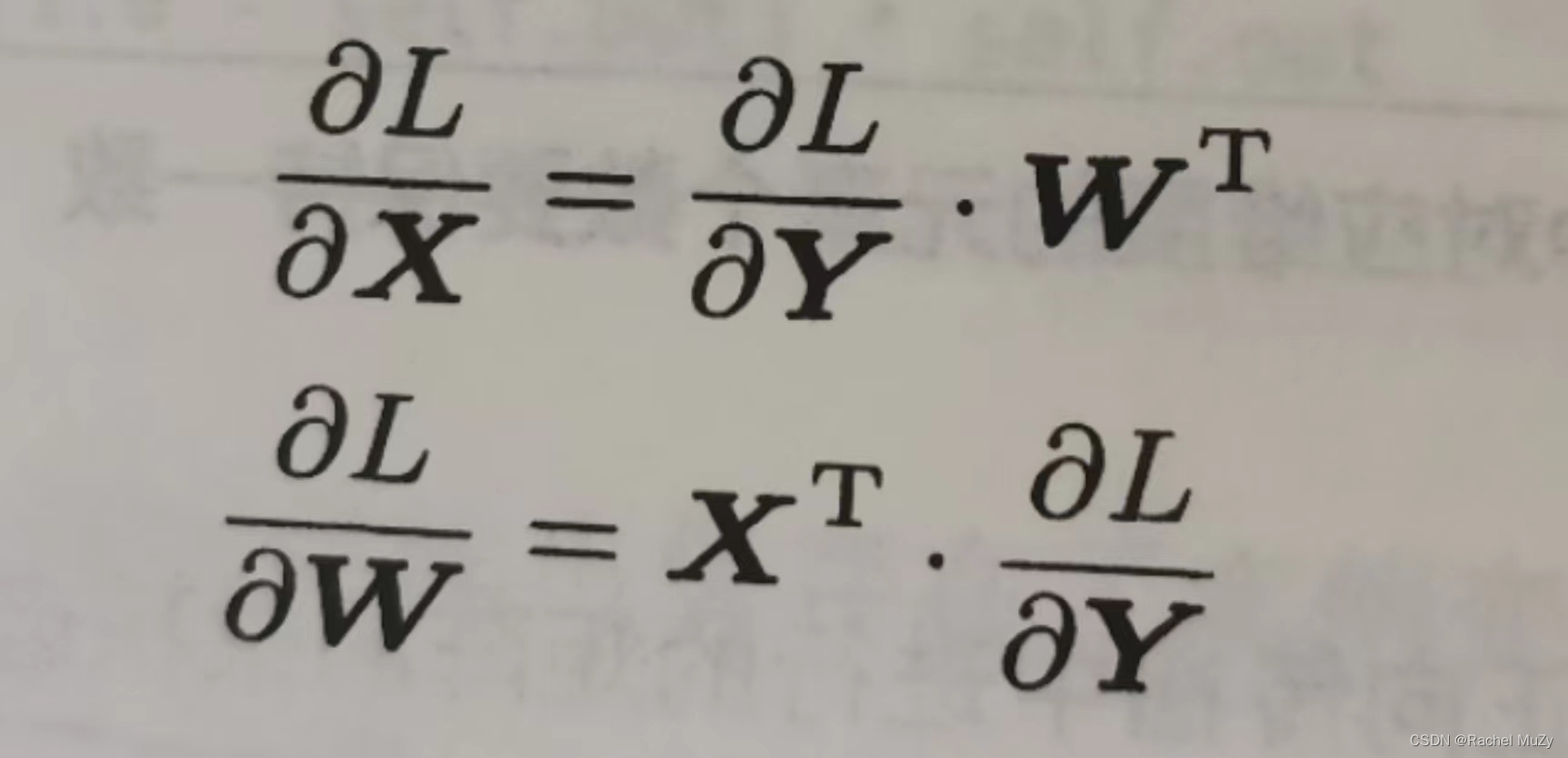https://zhuanlan.zhihu.com/p/349469914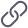https://zhuanlan.zhihu.com/p/349469914 前面介绍的Affine层的输入 X 是以单个数据为对象的，现在我们考虑N个数据一起进行正反向传播的情况，即批版本的Affine层。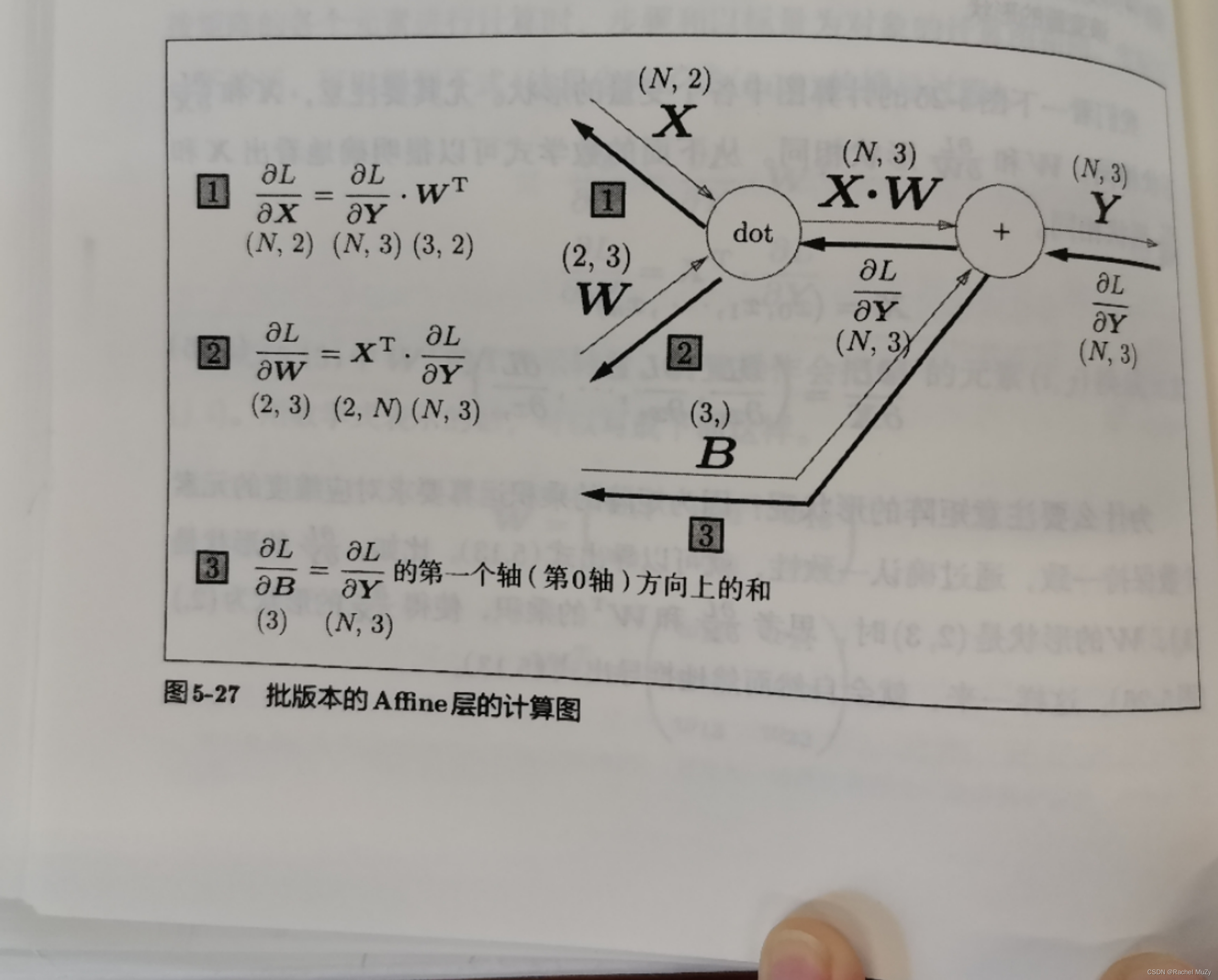``````import numpy as np

#forward
X_DOT_W = np.array([[0, 0, 0], [10, 10, 10]])
B = np.array([1, 2, 3])
Y1 = X_DOT_W + B
print(Y1)

#backward
dY = np.array([[1, 2, 3], [4, 5, 6]])
dB = np.sum(dY, axis = 0)
print(f'dB value is {dB}')``````

``````[[ 1  2  3]
[11 12 13]]
dB value is [5 7 9]``````

``````import numpy as np

class Affine:
def __init__(self, W, b):
self.W = W
self.b = b
self.X = None
self.dW = None
self.db = None

def forward(self, x):
self.x = x
out = np.dot(x, self.W) + self.b
return out

def backward(self, dout):
#dout默认的是L对Y的求导
dx = np.dot(dout, self.W.T)
self.dW = np.dot(self.W.T, dout)
self.db = np.sum(dout, axis = 0)``````